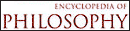# Boole - Research Article from Encyclopedia of Philosophy

This encyclopedia article consists of approximately 3 pages of information about Boole.
 This section contains 609 words (approx. 3 pages at 300 words per page) View a FREE sampleGeorge Boole (1815–1864) was the founder of modern mathematical logic. Nevertheless, few of his ideas are currently accepted in mainstream logic in the forms originally proposed by him. His learned and fertile mind conceived of several important hypotheses, the testing and modification of which changed the face of logic irrevocably. One of his most important hypotheses was that every proposition can be expressed using an algebraic equation suitably reinterpreted: that logic and algebra share a common uninterpreted formal language and thus also that they have similar problem types and similar methods.

The universal affirmative, or A proposition, "Every square is a rectangle" was expressed by x = xy, where x is the class of squares, y the class of rectangles, and xy the "Boolean or logical product" of x with y, the class of common members of x and y. The universal negative, or E proposition, "No rectangle is a...

 This section contains 609 words (approx. 3 pages at 300 words per page) View a FREE sample